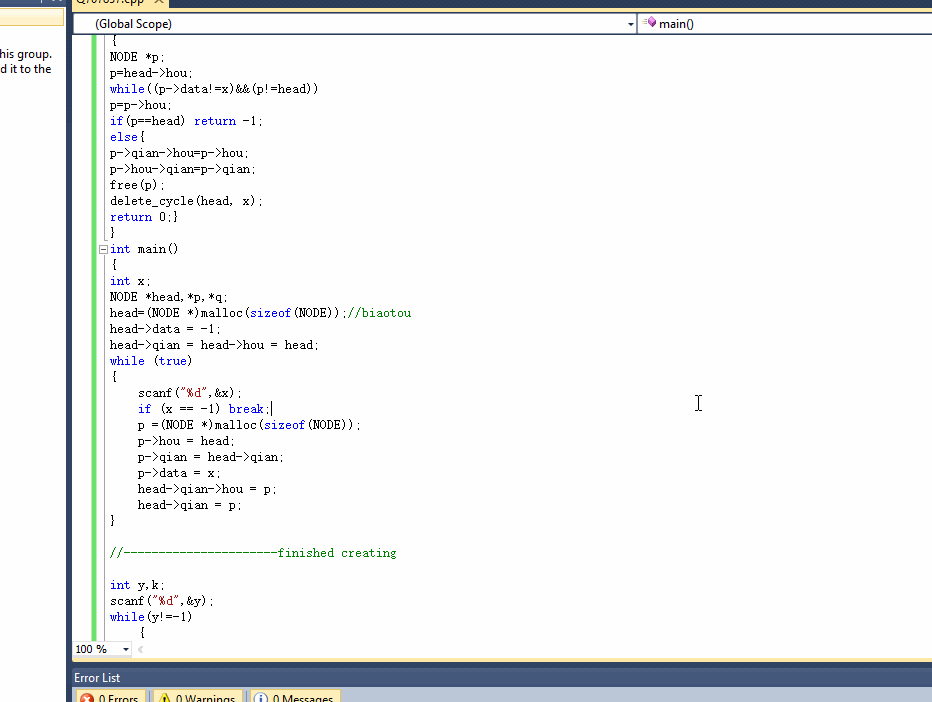Input

Output

Examples
Input

5
1
1
3
2
5
4
3
3
4
-1
3
2
6
-1

Output

-1
5 1 1 5 4 4

Note

## 只需在最后输出链表元素，空格分隔

#include
#include
#include
typedef struct node
{
int data;
struct node *qian,*hou;
} NODE;
{
NODE *p;
p=p->hou;
else{
p->qian->hou=p->hou;
p->hou->qian=p->qian;
free(p);
return 0;}
}
int main()
{
int x;
scanf("%d",&x);
if(x!=-1)
{
q=(NODE *)malloc(sizeof(NODE));
q->data=x;
p=q;
}
else
{
}
getchar();
scanf("%d",&x);
while(x!=-1)
{
q=(NODE *)malloc(sizeof(NODE));
q->data=x;
p->hou=q;
q->qian=p;
p=q;
scanf("%d",&x);
}
//chuxian -1

``````//----------------------finished creating

int y,k;
scanf("%d",&y);
while(y!=-1)
{
if(k==-1)
printf("-1\n");
scanf("%d",&y);
}
NODE *pr;
do
{
printf("%d ",pr->data);
pr=pr->hou;
``````

}

2个回答

``````#include "stdio.h"
#include "stdlib.h"
#include "string.h"

typedef struct node
{
int data;
struct node *qian,*hou;
} NODE;
{
NODE *p;
p=p->hou;
else{
p->qian->hou=p->hou;
p->hou->qian=p->qian;
free(p);
return 0;}
}
int main()
{
int x;
while (true)
{
scanf("%d",&x);
if (x == -1) break;
p =(NODE *)malloc(sizeof(NODE));
p->data = x;
}

//----------------------finished creating

int y,k;
scanf("%d",&y);
while(y!=-1)
{
if(k==-1)
printf("-1\n");
scanf("%d",&y);
}
NODE *pr;
do
{
printf("%d ",pr->data);
pr=pr->hou;
}

````````````在主函数里调用delete_cycle      时出错了，把x改成y
``````
`````` k = delete_cycle(head, y);
``````
``````还有就是delete_cycle  函数也有问题，你只删除了一个元素，题目要求删除所有相同的元素
``````